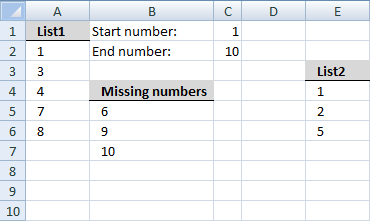Author: Oscar Cronquist Article last updated on August 12, 2018

### Question:

I want to find missing numbers in two ranges combined? They are not adjacent.

### Answer:### Array formula in cell B5:

=SMALL(IF((COUNTIF(List1, ROW(INDEX(\$A:\$A, \$F\$2):INDEX(\$A:\$A, \$F\$3)))+COUNTIF(List2, ROW(INDEX(\$A:\$A, \$F\$2):INDEX(\$A:\$A, \$F\$3))))=0, ROW(INDEX(\$A:\$A, \$F\$2):INDEX(\$A:\$A, \$F\$3)), ""), ROW(A1)))

How to create an array formula

1. Select cell B5
2. Click in formula bar3. Copy and paste array formula to formula bar
4. Press and hold Ctrl + Shift
5. Press Enter
6. Release all keys

### To remove #NUM errors, Excel 2007 users can use this formula:

=IFERROR(SMALL(IF((COUNTIF(List1, ROW(INDEX(\$A:\$A, \$F\$2):INDEX(\$A:\$A, \$F\$3)))+COUNTIF(List2, ROW(INDEX(\$A:\$A, \$F\$2):INDEX(\$A:\$A, \$F\$3))))=0, ROW(INDEX(\$A:\$A, \$F\$2):INDEX(\$A:\$A, \$F\$3)), ""), ROW(A1)), "")

### Named ranges

List1 (A2:A6)
List2 (E4:E6)
missing_list_start (B5)
What is named ranges?

### Explaining array formula in cell B5

=SMALL(IF((COUNTIF(List1, ROW(INDEX(\$A:\$A, \$F\$2):INDEX(\$A:\$A, \$F\$3)))+COUNTIF(List2, ROW(INDEX(\$A:\$A, \$F\$2):INDEX(\$A:\$A, \$F\$3))))=0, ROW(INDEX(\$A:\$A, \$F\$2):INDEX(\$A:\$A, \$F\$3)), ""), ROW(A1)))

Step 1 - Create dynamic array with numbers from start to end

=SMALL(IF((COUNTIF(List1, ROW(INDEX(\$A:\$A, \$F\$2):INDEX(\$A:\$A, \$F\$3)))+COUNTIF(List2, ROW(INDEX(\$A:\$A, \$F\$2):INDEX(\$A:\$A, \$F\$3))))=0, ROW(INDEX(\$A:\$A, \$F\$2):INDEX(\$A:\$A, \$F\$3)), ""), ROW(A1)))

ROW(INDEX(\$A:\$A, \$F\$2):INDEX(\$A:\$A, \$F\$3))

becomes

ROW(INDEX(\$A:\$A, 1):INDEX(\$A:\$A, 10))

becomes

ROW(\$A\$1:INDEX(\$A:\$A, 10))

becomes

ROW(\$A\$1:\$A\$10)

and returns this array:

{1; 2; 3; 4; 5; 6; 7; 8; 9; 10}

Step 2 - Find missing numbers

=SMALL(IF((COUNTIF(List1, ROW(INDEX(\$A:\$A, \$F\$2):INDEX(\$A:\$A, \$F\$3)))+COUNTIF(List2, ROW(INDEX(\$A:\$A, \$F\$2):INDEX(\$A:\$A, \$F\$3))))=0, ROW(INDEX(\$A:\$A, \$F\$2):INDEX(\$A:\$A, \$F\$3)), ""), ROW(A1)))

(COUNTIF(List1, ROW(INDEX(\$A:\$A, \$F\$2):INDEX(\$A:\$A, \$F\$3)))+COUNTIF(List2, ROW(INDEX(\$A:\$A, \$F\$2):INDEX(\$A:\$A, \$F\$3))))

becomes

(COUNTIF(List1, {1; 2; 3; 4; 5; 6; 7; 8; 9; 10})+COUNTIF(List2, {1; 2; 3; 4; 5; 6; 7; 8; 9; 10}))

becomes

(COUNTIF({1;3;4;7;8}, {1; 2; 3; 4; 5; 6; 7; 8; 9; 10})+COUNTIF({1;2;5}, {1; 2; 3; 4; 5; 6; 7; 8; 9; 10}))

{0;1;1;0;0;1;1;0;0} + {1;0;0;1;0;0;0;0;0}

and returns

{1;1;1;1;0;1;1;0;0}

Step 3 - Convert boolean array into missing numbers

=SMALL(IF((COUNTIF(List1, ROW(INDEX(\$A:\$A, \$F\$2):INDEX(\$A:\$A, \$F\$3)))+COUNTIF(List2, ROW(INDEX(\$A:\$A, \$F\$2):INDEX(\$A:\$A, \$F\$3))))=0, ROW(INDEX(\$A:\$A, \$F\$2):INDEX(\$A:\$A, \$F\$3)), ""), ROW(A1)))

IF((COUNTIF(List1, ROW(INDEX(\$A:\$A, \$F\$2):INDEX(\$A:\$A, \$F\$3)))+COUNTIF(List2, ROW(INDEX(\$A:\$A, \$F\$2):INDEX(\$A:\$A, \$F\$3))))=0, ROW(INDEX(\$A:\$A, \$F\$2):INDEX(\$A:\$A, \$F\$3)), "")

becomes

IF(({1;1;1;1;0;1;1;0;0}, ROW(INDEX(\$A:\$A, \$F\$2):INDEX(\$A:\$A, \$F\$3)), "")

becomes

IF(({1;1;1;1;0;1;1;0;0}, {1; 2; 3; 4; 5; 6; 7; 8; 9; 10}, "")

and returns

{"";"";"";"";6;"";"";9;10}

Step 4 - Return the k-th smallest number

=SMALL(IF((COUNTIF(List1, ROW(INDEX(\$A:\$A, \$F\$2):INDEX(\$A:\$A, \$F\$3)))+COUNTIF(List2, ROW(INDEX(\$A:\$A, \$F\$2):INDEX(\$A:\$A, \$F\$3))))=0, ROW(INDEX(\$A:\$A, \$F\$2):INDEX(\$A:\$A, \$F\$3)), ""), ROW(A1)))

becomes

=SMALL({"";"";"";"";6;"";"";9;10}, ROW(A1))

becomes

=SMALL({"";"";"";"";6;"";"";9;10}, 1)

returns the number 6 in cell B5.

### How to customize the formula to your excel spreadsheet

Change the named ranges.

### Download excel sample file for this tutorial.

missing-values-in-two-columns.xlsx
(Excel 2007 Workbook *.xlsx)